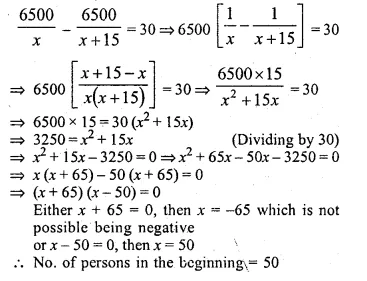Guru

# (i) Rs. 480 is divided equally among ‘x’ children. If the number of children was 20 more then each would have got Rs. 12 less. Find ‘x’. (ii) Rs. 6500 is divided equally among a certain number of persons. Had there been 15 more persons, each would have got Rs. 30 less. Find the original number of persons.

• 0

This question has been taken from Book:- ML aggarwal, Avichal publication, class10th, quadratic equation in one variable, chapter 5, exercise 5.5
This is an important ques

(i) Rs. 480 is divided equally among ‘x’ children. If the number of children was 20 more then each would have got Rs. 12 less. Find ‘x’. (ii) Rs. 6500 is divided equally among a certain number of persons. Had there been 15 more persons, each would have got Rs. 30 less. Find the original number of persons.
Question no34. , ML Aggarwal, chapter 5, exercise 5.5, quadratic equation in one variable, ICSE board,

Share